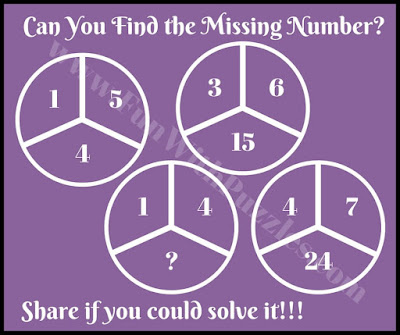## Thursday, August 31, 2017

Its long time we have published Maths Brain Teasers. Our previous post on Maths Puzzle was "Interesting and Confusing Math Brain Test" which contained different Maths Puzzles which were used with different geometrical shapes. E.g. it contained Maths Puzzles with Square Shape in which four number were given. It also contained Three Squared Math Puzzles in which 3 numbers were given. Also it contained Maths Brain Teaser in Triangular as well as Circle Shapes. One thing was common in all these Math Brain Teasers is that it contain certain set of numbers which share a relationship among each other. One has to find this relationship among these numbers and then solve it for the missing number.
In these Cool Maths Quiz for Kids, four circles are given. Each of the circle contains three numbers. These three numbers share one Mathematical relationship with each other. One has to find this pattern or relationship among these three numbers and then has to solve each Maths Brain Teaser for the missing number which will replace the question mark.1. What will replace the question mark?2. Calculate value of Missing Number?3. What is the value of Missing Number?4. Can you solve this Simple Maths Puzzle?5. Solve this Maths Riddle to find value of missing number?
If you love to solve Maths Brain Teaser, then you can checkout the following Maths Puzzles.

Answers of these Cool Maths Quiz Brain Teasers is given as below

1. 4

2. 17

3.  2

4.  3

5. 10

1.1.